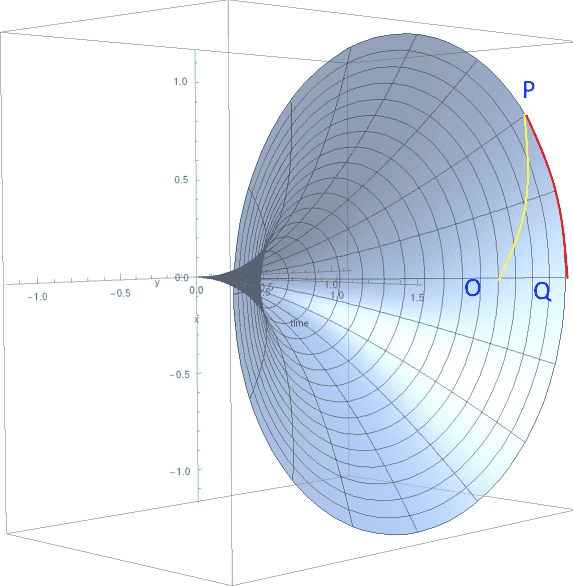# The Distance between two points in a hypothetical universe

• A
I have a hypothetical universe where the distance between two points in spacetime is defined as:
$$ds^2 =−(\phi^2 t^2)dt^2+dx^2+dy^2+dz^2$$Where ##\phi## has units of ##km s^{-2}##. The space in this universe grows quadratically with time (and, as I understand it, probably isn’t Minkowski space). A particle travelling at the speed of causality, c, will follow this contour from point O to point P.Given the time between point O and point Q, is it possible to find the distance from point Q to point P (e.g. does a function exist such that ##f(\Delta t) = d_L##). If so, what is the formula?

#### Attachments

Last edited:

Orodruin
Staff Emeritus
Homework Helper
Gold Member
2021 Award
What you have written down is just the line element of Minkowski space in different coordinates. Is it what you intended to write or did you intend it to be a RW universe?

The space in this universe grows quadratically with time (and, as I understand it, probably isn’t Minkowski space).
"Space" is relative and not something that is uniquely defined for a spacetime. It is a concept that belongs to the coordinates. See my PF Insight on the topic. Furthermore, "space" can never be Minkowski space as Minkowski space is a 4-dimensional spacetime.

Given the time between point O and point Q, is it possible to find the distance from point Q to point P (e.g. does a function exist such that f(Δt)=dLf(Δt)=dLf(\Delta t) = d_L). If so, what is the formula?
Is the question you intended to ask "how can I find the proper distance to the point where the light signal is after a time ##\Delta t## (with the usual caveats on what this means). Note that this is not a luminosity distance (which is what is usually denoted ##d_L##. Also, your figure shows an expanding circle, not an expanding flat space as in your metric (if corrected to be a RW metric).

Edit: Additionally, note that even if you move the scale factor to the spatial part your universe will not grow quadratically. The RW metric scale factors enters squared in the line element and so your universe would grow linearly. Also note that if you move your current scale factor to the spatial oart, then you have a coasting universe, which is again just Minkowski space in different coordinates.